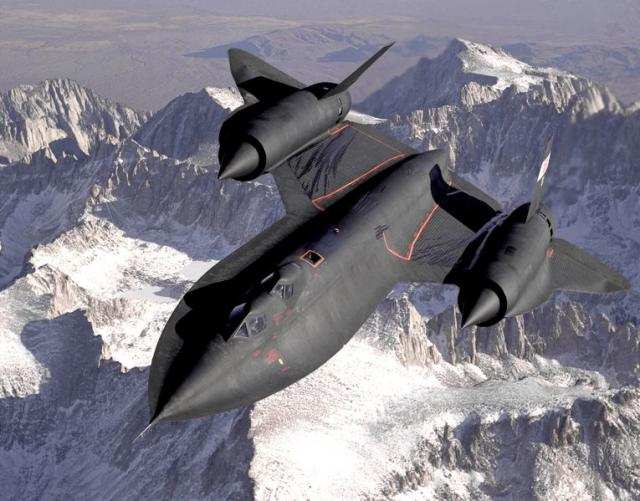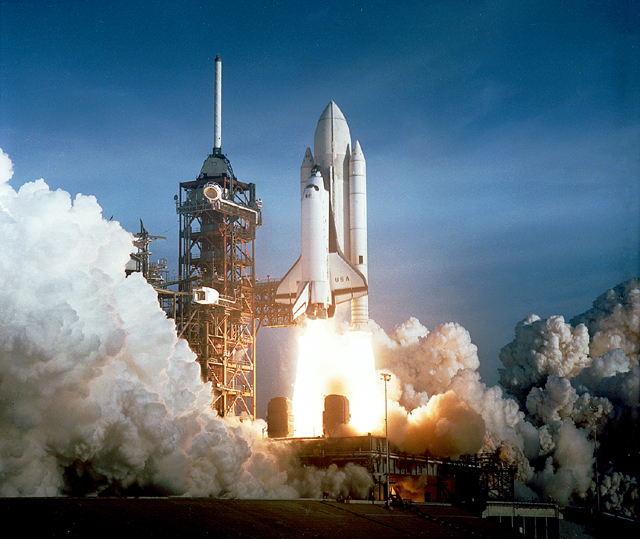# Mach Number

The dimensionless Mach number is a means to represent the speed of an object through a fluid (or the speed of a fluid over an object) relative to the speed of sound in the fluid.

More formally, the Mach number is defined as:

M = V / a

where:
M is the dimensionless Mach number
V is the fluid or object speed
a is the speed of sound in the fluid

For an ideal gas (such as air, when not under extremely low or high temperatures):

a2 = gamma . R . T / W

where:
R is the universal gas constant
T is the temperature
W is the molar mass
gamma is the heat capacity ratio or adiabatic index

gamma = CP / CV

where:
CP is the heat capacity at constant pressure
CV is the heat capacity at constant volume

The speed of sound in air at sea level is 344m/s (1238km/h or 769.5mph) and is calculated using:
R = 8.314472 J · K-1 · mol-1
T = 293.15oK (20oC or 68oF)
W = 0.02896 Kg · mol-1
gamma = 1.403Lockheed SR71 Blackbird: Capable of Mach 3Image courtesy of NASA Dryden

Flow regimes can be characterized according to Mach number as:

• Incompressible subsonic flow (M < 0.3) – within this range gases (e.g. air) behave like incompressible fluids (e.g. water). Insects, birds, gliders, small propeller airplanes, helicopters and cars operate within such air speeds.
• Compressible subsonic flow (0.3 < M < 1) – most passenger jetliners operate within this speed range and more specifically within what is known as transonic flow (0.7 < M < 1.2). Transonic flow incorporates regions of supersonic and subsonic flow separated by weak shockwaves. As an airplane approaches the sound barrier (M = 1) the drag increases significantly and then subsides once the barrier is exceeded.
• Supersonic flow (M > 1) – is characterized by strong shock waves that attach to sharp geometric features such as wing trailing edges. Concorde (M = 2) and the Lockheed SR71 Blackbird (M = 3) were rare examples of airplanes that could sustain supersonic speeds for long durations. While still supersonic, hypersonic flow (M > 5) is the term used to designate speeds that are typical of missiles and spacecraft entering a planet's atmosphere. The Space Shuttle Orbiter encounters M = 25 as it re-enters the Earth's atmosphere from orbit. These speeds produce extremely high temperatures, enough to ionize and dissociate gas molecules. Specially shaped nozzles called de Laval nozzles can also generate hypersonic fluid flow at sea level.Space Shuttle on Re-entry Reaches M = 25Image courtesy of NASA